Next: Example of Step by Up: Using the Y-Combinator to Previous: Introduction   Contents   Start

#### Steps to Eliminate Implicit Recursion

1. recursive function to calculate the factorial including illegal implicit recursion: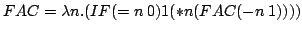2. locating illegal implicit recursion: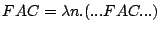3. eliminating illegal implicit recursion: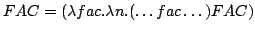4. simplified representation: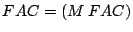where: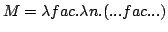The recursion is eliminated by enclosing the recursive function in a lambda abstraction, that receives the function to be invoked recursively as an argument.

5. Using definition 12 and the result in step 4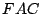is identified as a fixed point of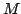.

Therefore we can write: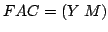6. The function to calculate the factorial can now be rewritten as: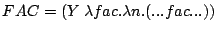7. To compute the factorial of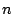the following expression has to be evaluated:

((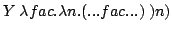Georg P. Loczewski 2004-03-05

Impressum und Datenschutz